Hello everyone,

Now it's summer, and some of you are already on vacation. It's time for some well-deserved rest. Personally, I love spending my holidays lazing around, reading books, and learning new things.

Last week, I decided to study an important branch of statistics: the theory of probability. We recently talked about descriptive statistics, so let's take another step. Are you ready? Well then, let's get started.

I quickly realized that I can't study the entire theory of probability because it's too vast and, in some ways, much more related to pure mathematics than statistics. As a bioinformatician, my goal is to familiarize myself as much as possible with the world of statistics so that I can interpret and understand the results correctly. Mastering the entire theory of probability requires a dedicated study path.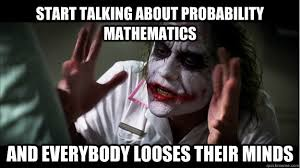So let me be clear, what is covered in this article is just a part of the theory of probability. The essentials, at least for now, to perform well in my work as a bioinformatician.

The theory of probability is that part of statistics that deals with the study of probability. Probability indicates the likelihood of a certain event occurring and is quantified with values ranging from 0 to 1. An event with a probability of 0 is impossible to happen, just as an event with a probability of 1 is certain to happen.

The concept underlying the theory of probability is that of random variable. A variable is a characteristic of a statistical unit under study. For example, the hair color of the student Ivar is a variable, and its value, in this case, is blonde, which is what we observe. During our statistical analysis, we may encounter variables whose value is not yet known because the experiment has not been conducted yet, the event we want to observe has not occurred yet, or because the sample has not been drawn, and we do not have statistical units to observe the variable's values. These variables whose value is not yet known at the time of the statistical study are called random variables.

Let's make an example of a random variable. Imagine flipping a coin. Before the flip, you do not know the outcome. Therefore, the variable "result of the flip" is random. This variable remains random until I toss the coin and observe the result of the event.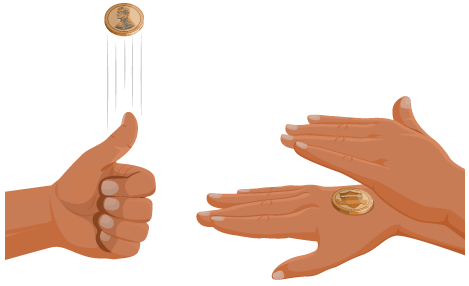The entire theory of probability revolves around the concept of random variable because its purpose is imagine or predict the value of a random variable simply through evaluations called "probability assessments."

Thanks to the theory of probability, we can predict the outcome of a coin flip before it happens, albeit with a certain degree of uncertainty. Pretty cool, right?

As mentioned, the probability of an event happening must be quantified, but to do that, we must first clarify what probability is. There are three definitions of probability, and each involves a different way of calculating probability:

• Classic definition of probability.
• Frequentist or empirical definition of probability.
• Subjective definition of probability.

The classic definition of probability states that to quantify the possibility of an event happening, we must take the ratio of the number of favorable cases (i.e., the number of cases in which the event can actually occur) to the number of possible cases (i.e., the total number of times the event can occur).

Let's take an example. Let's say we want to predict the outcome of flipping a coin.

The variable "outcome of the coin flip" has two favorable values: heads and tails. The number of possible cases in this scenario is 1. Indeed, we want to predict the value of a single coin flip.

Thus, according to the classic definition of the probability of an event, we will have:

``P(event) = number of favorable cases / number of possible cases``

In this example, the number of favorable cases is 1 (as it can only be heads or tails), while the number of possible cases is 2 (as there are two possible outcomes of the flip). Therefore, the probability of getting heads or tails is 1/2.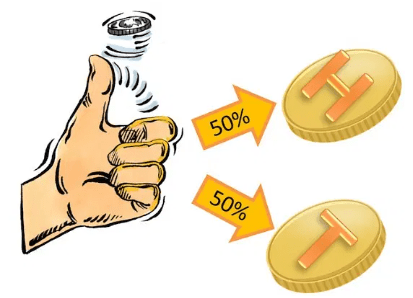In the frequentist definition of probability, it is stated that:

"For an infinite (or very large) number of trials, the probability that an event occurs tends to its true value, which is the relative frequency value of the event recorded after that number of trials."

Feeling a bit confused? Don't worry, let's provide an example right away:

Let's say we have a black box in which, we have been told, there are blue and red balls. Now, the question is: what is the probability of drawing a red ball?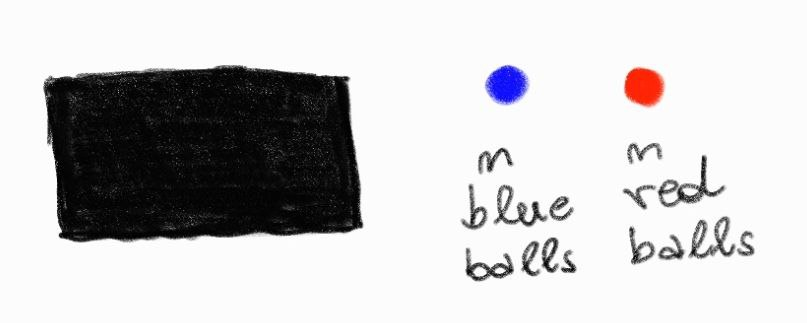To answer this question, we can apply exactly what the frequentist method of calculating probability states. We can draw a ball from the black box a very large number of times and record each observation. Then, we can apply the classic formula for calculating probability to the obtained numbers. This is an empirical way of calculating probability, but with confidence in the frequentist method and the large number of experiments conducted, we can consider this probability value to be the one closest to reality. Therefore, we can admit that the most frequent value is the most probable one since, according to the frequentist definition, the probability of an event tends to be equal to the relative frequency with which it occurred during the numerous experiments.

Before moving on to the subjective definition of probability, I would like to make some considerations:

• The calculation of probability using the frequentist method is purely empirical and therefore not demonstrable. Additionally, this method requires that the phenomena studied during the recording of relative frequencies are repeatable and regular over time.
• In the classical conception, probability is calculated A PRIORI, before looking at the data, whereas in the frequentist conception, probability is derived A POSTERIORI, i.e., after examining the data.
• If we indicate with "p" the probability that an event occurs, the contrary probability, i.e., that the event does not occur, is indicated as "q," and it is equal to q = 1 - p, since q + p = 1.

Now let's talk about the subjective definition of probability. It is important to clarify from the beginning that this definition is not at all rigorous and scientific precisely because it is subjective and therefore not objective.

According to this definition, the probability that an event occurs depends on how much an individual, with conviction, judges a certain event under consideration to be possible.

Applying the subjective definition of probability is equivalent to talking with friends at the bar and stating that, in our opinion, Juventus has a 60% probability of winning the championship. That probability percentage has been decided in a non-rigorous and scientific way, solely based on our belief in whether Juventus can win the championship or not.

So, to reiterate, we cannot fully rely on the subjective definition of probability, but sometimes this is the only type of probability we can refer to.

Let's think again about the random variable: "Juventus winning the championship." To calculate the probability that Juventus wins the championship in 2023/2024, we cannot resort to the classic definition of probability, as the classic probability is calculated as favorable events over total events. We immediately notice that there are no multiple possible events. In fact, the favorable event is 1 (i.e., Juventus wins the championship), and the possible event is also 1, since there are no multiple 2023/2024 championships; it is only played in that year. Applying the classic definition, the probability that Juventus wins the championship in 2023/2024 is 100%, but does it seem like a certainty that Juventus will win? If that were the case, it wouldn't make sense to play.

Likewise, we cannot apply the frequentist definition of probability because we cannot record the frequencies of Juventus winning the championship in 2023/2024. The annual championship is played only once a year; we cannot ask the different teams to play the championship matches multiple times in a year.

In short, in cases like these, where it is not possible to apply either the classic or the frequentist definition of probability, we have no choice but to rely on our subjective judgment to describe the probability that an event occurs.

But is it possible to mathematically calculate probability according to the subjective definition? Well, with a little stretch, yes, and we must thank an Italian mathematician for this, Bruno De Finetti (1906-1985).

De Finetti gave an operational definition for subjective probability, stating that:

"The subjective probability of an event E, according to the opinion of a specific individual, is equal to the price that he considers fair to pay (C) to receive an amount (S) when the event E occurs."

So, in accordance with De Finetti, all we need to do is ask ourselves how confident we are that Juventus can win the championship and express this quantity in terms of money we are willing to bet to win 100 euros. Dividing these two quantities will give us a value, obviously not rigorous, of probability. See the image below:Well, we have talked about how we can calculate the probability of an event based on which definition of probability we choose to use. But until now, we have considered simple cases where we want to know the probability of a single event. We have answered questions like: "What is the probability of getting heads in a non-rigged coin toss?"

But what if I asked you a more complex question? Like: "What is the probability of getting two consecutive heads after two tosses of an unbiased coin?"

To answer these questions, we need to take a further step, but don't worry, I will show you some concrete examples right away.

Total Probability:

Let's suppose we have a deck of poker cards (52 cards in total) and we want to calculate the probability that, by drawing a card at random, we get an ace of hearts instead of an ace of diamonds. We are therefore asking ourselves what is the probability that only one of two considered events happens.

"Do the two events mutually exclude each other?"

• If the answer is yes, we define the two events as incompatible.
• If the answer is no, we define the two events as compatible.

In our specific case, the events are incompatible. Indeed, if we draw the ace of hearts, we did not draw the ace of diamonds, and vice versa. The two events exclude each other.

Once we have answered this first question, we can calculate the probability that only one of two considered events happens. We simply add the probabilities of the two individual events, thus obtaining the total probability. See image.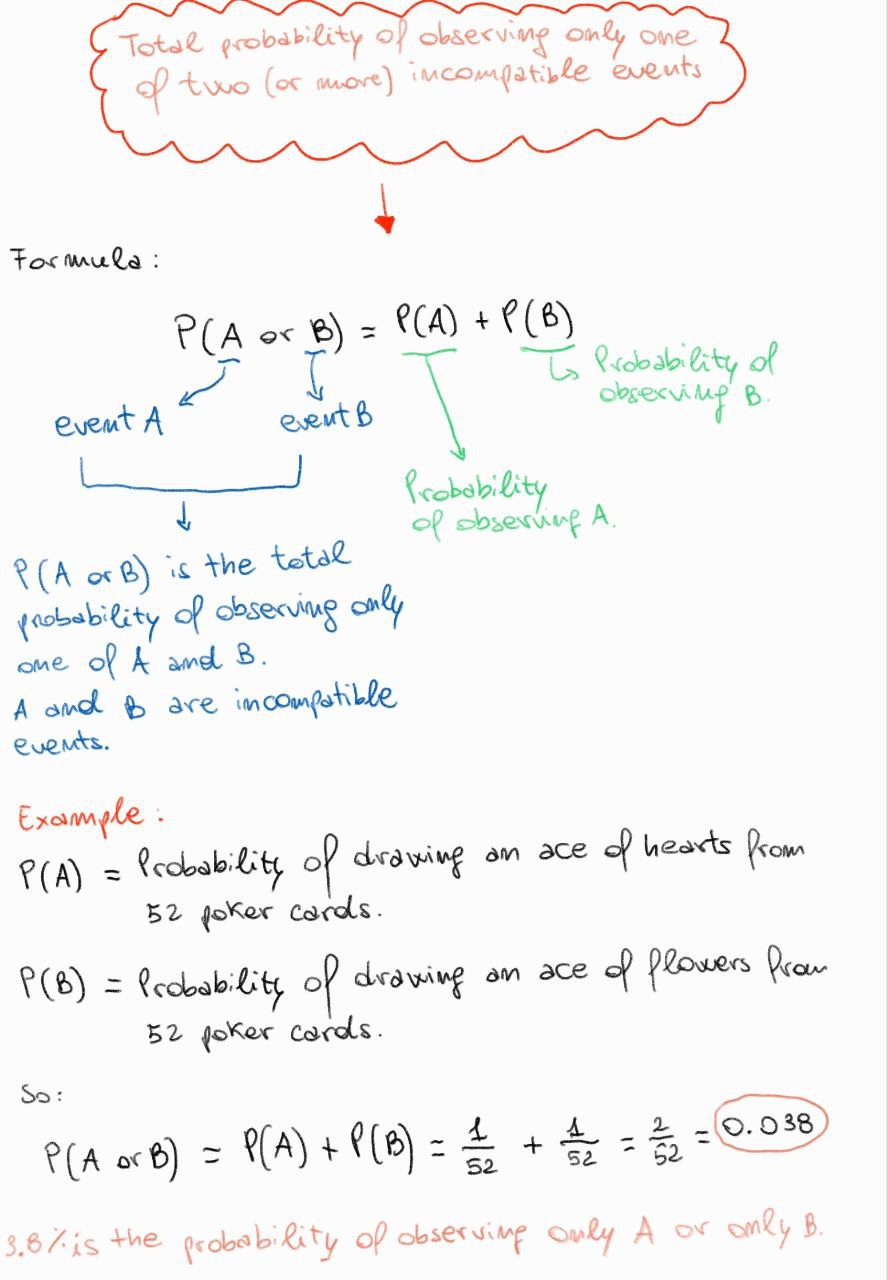Yes, I can hear you. I can hear you asking: "Why is it so important to ask whether the two considered events mutually exclude each other or not?"

This is important because it changes something in the calculation of the total probability. Let's do another example to understand what changes.

Using the same deck of poker cards, let's ask ourselves what is the probability of drawing an ace or a heart card. In this case, the two events do not mutually exclude each other since I could draw an ace of hearts and satisfy both events, and therefore, these events are compatible. Thus, in this case, the total probability must also take into account the case where both events are observed when we want to know the probability that only one of two considered events happens.

See the image below to understand better.Composite Probability:

Let's take the deck of poker cards again. Now we wonder what is the probability of drawing three aces in a row. In other words, we are asking ourselves what is the probability of observing two or more consecutive events, no longer one instead of the other, but one after the other.

The probability that two or more events occur in succession is called composite probability, which is obtained by multiplying the probabilities of the individual events.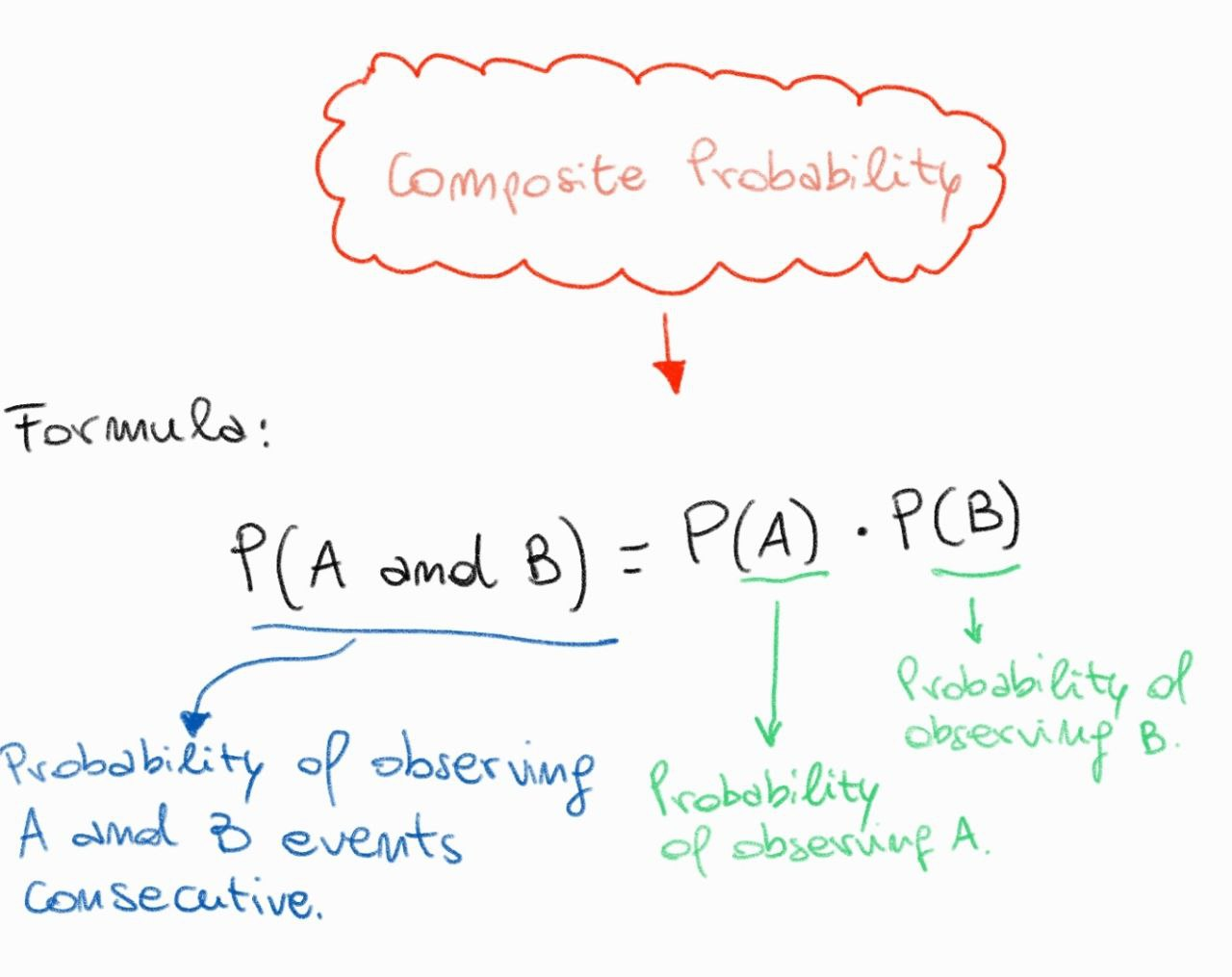But, as with total probability, to calculate the composite probability, we must first ask a question:

"After observing an event, is the starting condition restored?"

• If the answer is yes, the events are independent.
• If the answer is no, the events are dependent. In this case we speak of conditional probability.

For example, if after drawing and observing the first card, I put it back in the deck, I will have a situation of independence between the observed events (Case 1). If I don't put the card back in the deck, it's obvious that the total number of cards will be lower (52 - 1 = 51 cards), and thus the probability will be different (Case 2).

Let's examine the two cases to understand the difference better: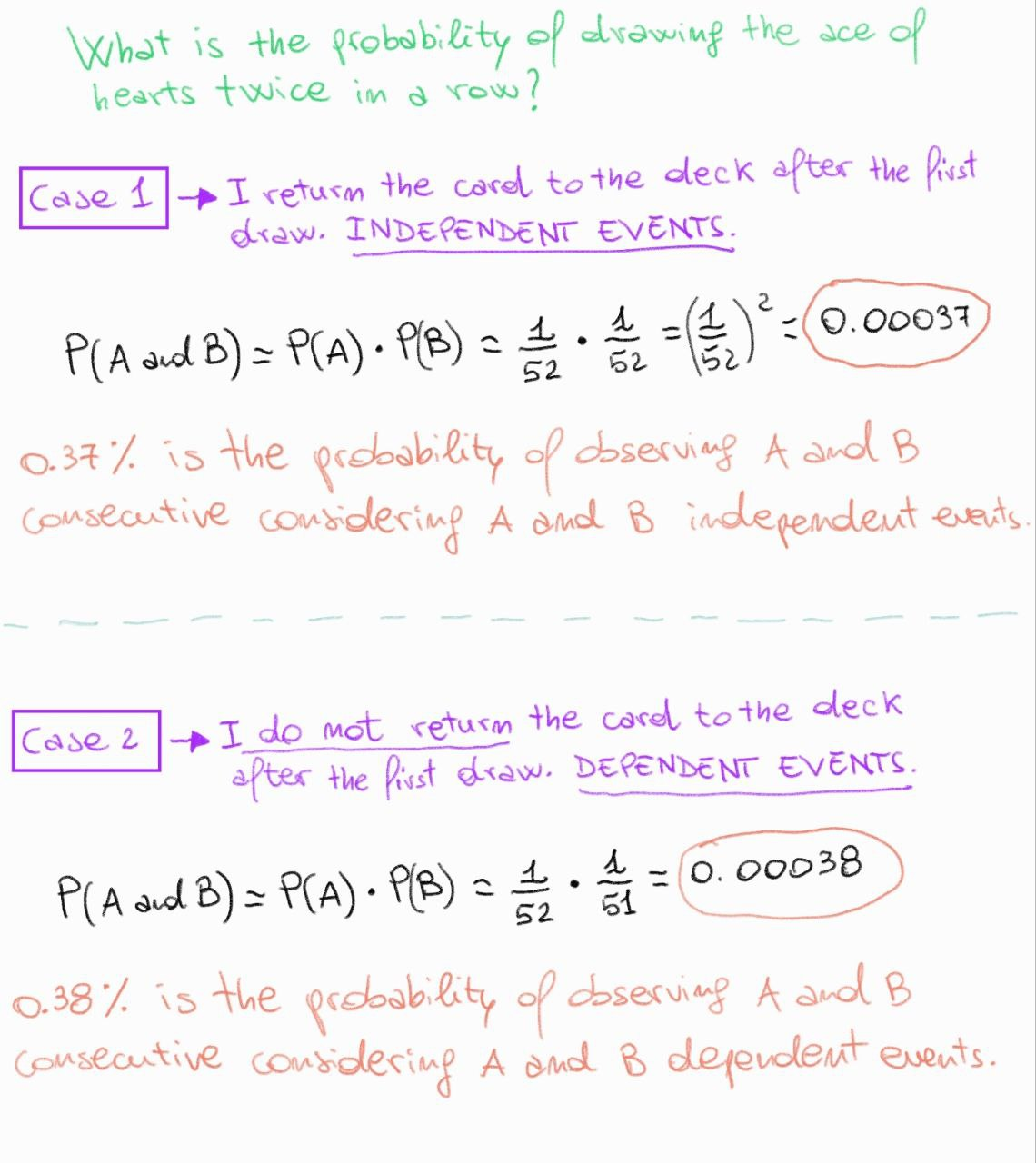Analyzing the example shown in the image above, we can make two important observations regarding the composite probability:

• The composite probability of observing consecutive events is higher if they are dependent and lower if they are independent.
• The greater the number of consecutive events, the lower the composite probability of observing them in succession.

Alright. I think I've given you enough information for today. In the next article, we will continue to talk about probability theory, and more specifically, how by plotting the calculated probabilities for each value that a certain random variable can take, we can build graphs, called probability distributions, which are the basis of the last branch of statistics we will cover, namely statistical inference.

As always, I ask you to leave a comment, even to make appropriate corrections, so that we can all make the best use of this popularization project.

Goodbye and see you soon!